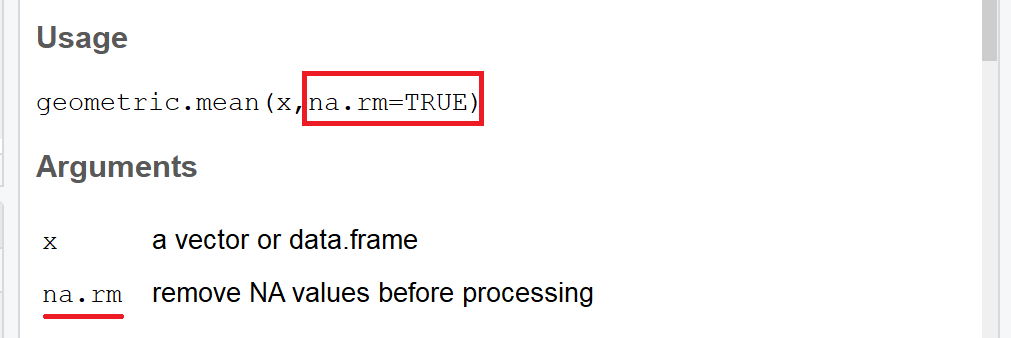# Geometric Mean in R (2 Examples)

This post explains how to compute the geometric mean in the R programming language.

So without further ado, let’s move on to the examples!

## Example Data

In the examples of this tutorial, I’ll use the following numeric vector as exemplifying data:

`x <- c(8, 9, 4, 1, 6, 4, 6, 2, 5)  # Create example data`

Of cause, we could also apply the following R codes to any other numeric vector or to a numeric column of a data frame.

However, let’s move on to the examples…

## Example 1: Compute Geometric Mean Manually

In the first example, we will compute the geometric mean manually based on the already built-in R functions exp(), mean(), and log(). We can calculate the geometric mean based on these R functions as follows:

```exp(mean(log(x)))                  # Compute geometric mean manually
# 4.209156```

As you can see, the geometric mean of our example data is 4.209156.

This seems to be too complicated to do it on a regular basis? No problem! The following example makes the computation of the geometric mean even easier…

## Example 2: geometric.mean Function of psych R Package

The psych package is an R add-on package which, besides many other very useful functions, contains the geometric.mean command. Let’s see how we can use the geometric.mean function in practice.

First, we need to install and load the psych package to R…

```install.packages("psych")          # Install psych package

…and then we can apply the geometric.mean function to compute the geometric average of our example data:

```geometric.mean(x)                  # Apply geometric.mean function
# 4.209156```

The result is 4.209156 – the same value we got with our manual solution of Example 1.

By the way: The geometric.mean function also provides an option to remove NA values from your computation of the geometric mean. Definitely an additional advantage of the psych package compared to the manual solution.Figure 1: Excerpt of Help Documentation of geometric.mean() R Function.

## Video, Further Resources & Summary

For more detailed information concerning the specific code of this article, please check out the below video on the Statistics Globe YouTube channel:

Please accept YouTube cookies to play this video. By accepting you will be accessing content from YouTube, a service provided by an external third party.If you accept this notice, your choice will be saved and the page will refresh.

This article explained how to compute geometric means in the R programming language. In case you want to learn more about the theoretical research concept of the geometric mean, you may have a look at the following video tutorial of the YouTube channel of patrickJMT:

Please accept YouTube cookies to play this video. By accepting you will be accessing content from YouTube, a service provided by an external third party.If you accept this notice, your choice will be saved and the page will refresh.

Furthermore, you could have a look at some of the other RStudio tutorials that I have published on this website. I have written already several tutorials on the mean in R:

I hope this tutorial provided what you were looking for. However, don’t hesitate to let me know in the comments section below, in case you have any comments or questions. Also, don’t forget to subscribe to my email newsletter for regular R updates directly to your mailbox.

Subscribe to the Statistics Globe Newsletter

•Munish Kohli
August 2, 2021 12:48 am

Thanks for the info. This is useful, but how to handle negative values while calculating geometric.mean(x) ? In this case it is not able to calculate. Will really appreciate your help.

•Emily
November 29, 2022 6:51 pm

How do I calculate Geometric Standard Deviation?

•December 14, 2022 10:49 am